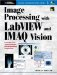# Pattern Matching

Pattern matching is arguably one of the most important image analysis tools and is very often the first step in a Machine Vision application. In general, pattern matching provides you with information about the position and the number of instances of a previously defined template (pattern).

Introduction

We can try a first pattern matching example in IMAQ Vision Builder. The procedure is similar to the previous shape matching example, with the main difference that the image has to be a gray-scaled one:

1. Define a template (ROI or file).

Figure 5.54. Pattern Matching with IMAQ Vision Builder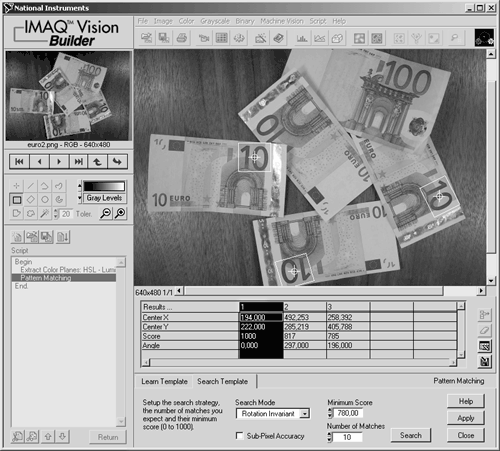Figure 5.54 also shows the parameters of the pattern matching process:

• the maximum number of instances that can be found;
• the selection between shift invariant and rotation invariant mode:
• Shift invariant mode does not allow rotation of potential matches in relation to the template; this means that only matches with the same orientation as the template are found.
• Rotation invariant allows matches in any direction; this mode is significantly slower than the shift invariant mode.

Figure 5.55 shows the result with the same image if the minimum matching score is decreased to 600; now the partly hidden 10 and the first two digits of the 100 note are found, although the 100 is a different color .

Figure 5.55. Pattern Matching: Decreasing Matching Score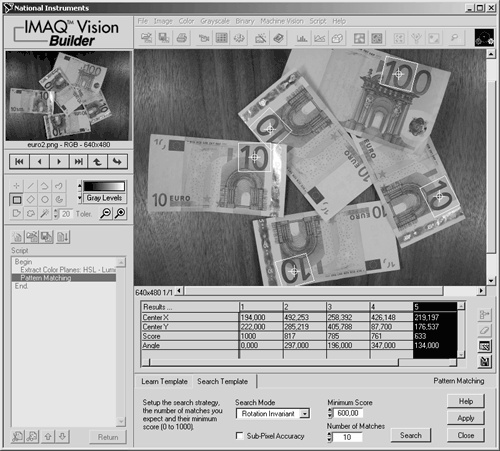Pattern Matching Techniques

The mathematical basis of pattern matching is the cross correlation function. The correlation C(i,j) is defined as

Equation 5.26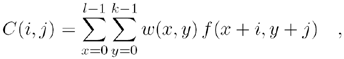where w ( x,y ) is a subimage (template) of the size k x l ; f ( x,y ) is the original image of the size m x n (where k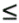m and ln ); i = 0, 1, ... m -1, j = 0, 1, ... n -1 .

Figure 5.56 shows the front panel of a LabVIEW VI provided on the attached CD-ROM. The program is similar to the IMAQ Vision Builder functionality and allows definition of a template and the matching process itself. Moreover, the orientation of the (first) match is displayed with a gauge control. See also Figure 5.57 for the diagram, showing the main loop of the pattern matching process.

Figure 5.56. Pattern Matching: Single MatchFigure 5.57. Part of the Pattern Matching DiagramThe same application finding multiple instances of the template can be seen in Figure 5.58. The Number ( # ) of Matches control has to be increased to the desired maximum number of instances. Note that the matches found in this example as well as in the Vision Builder are not scale invariant, which means that all instances have to be the same size as the template.

Figure 5.58. Pattern Matching: Multiple Match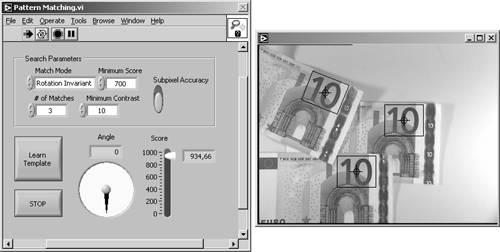Another problem with Eq. (5.26) is its high sensitivity to amplitude changes of the template as well as of the image, which has to be searched for matches. Amplitude changes are caused by different lighting conditions of new images or by brightness changes of the original image.

A normalized correlation coefficient R ( i,j ), as in

Equation 5.27solves this problem: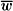is the average intensity value of the pixels in the template w , and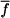is the average intensity value of the coincident image area f , which leads to a range for the value of R from “1 to 1. R is therefore independent of intensity changes of f and w .

The result of the function IMAQ Match Pattern is a data set for all matches found in the original image. In particular, the center coordinates and the rotation angle (in the case of rotation invariant matching) can be used for further image analysis, for example, for the definition of a new coordinate system.

IMAQ Vision also contains functions for color pattern matching, for example, IMAQ Color Match Pattern . These functions combine the gray-level pattern matching functions with the matching of color information. The results of both algorithms are used to calculate the total matching score. You can try these functions, using IMAQ Vision Builder.